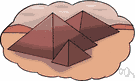# polyhedron

(redirected from Googolhedron)
Also found in: Thesaurus, Encyclopedia.

## pol·y·he·dron

(pŏl′ē-hē′drən)
n. pl. pol·y·he·drons or pol·y·he·dra (-drə)
A solid bounded by polygons.

## polyhedron

(ˌpɒlɪˈhiːdrən)
n, pl -drons or -dra (-drə)
(Mathematics) a solid figure consisting of four or more plane faces (all polygons), pairs of which meet along an edge, three or more edges meeting at a vertex. In a regular polyhedron all the faces are identical regular polygons making equal angles with each other. Specific polyhedrons are named according to the number of faces, such as tetrahedron, icosahedron, etc
[C16: from Greek poluedron, from poly- + hedron side, base]

## pol•y•he•dron

(ˌpɒl iˈhi drən)

n., pl. -drons, -dra (-drə).
a solid figure having many faces.
[1560–70; < Greek polýedron, neuter of polýedros having many bases. See poly-, -hedron]

## pol·y·he·dron

(pŏl′ē-hē′drən)
A three-dimensional geometric figure whose sides are polygons. A tetrahedron, for example, is a polyhedron having four triangular sides.
ThesaurusAntonymsRelated WordsSynonymsLegend:
 Noun 1polyhedron - a solid figure bounded by plane polygons or facessolid - a three-dimensional shapeconvex polyhedron - a polyhedron any plane section of which is a convex polygonconcave polyhedron - a polyhedron some of whose plane sections are concave polygonsprism - a polyhedron with two congruent and parallel faces (the bases) and whose lateral faces are parallelogramspyramid - a polyhedron having a polygonal base and triangular sides with a common vertextetrahedron - any polyhedron having four plane facespentahedron - any polyhedron having five plane faceshexahedron - any polyhedron having six plane facesoctahedron - any polyhedron having eight plane facesdecahedron - any polyhedron having ten plane facesdodecahedron - any polyhedron having twelve plane facesicosahedron - any polyhedron having twenty plane facesideal solid, Platonic body, Platonic solid, regular convex polyhedron, regular convex solid, regular polyhedron - any one of five solids whose faces are congruent regular polygons and whose polyhedral angles are all congruentprismatoid - a polyhedron whose vertices all lie in one or the other of two parallel planes; the faces that lie in those planes are the bases of the prismatoidtrapezohedron - a polyhedron whose faces are trapeziums
Translations
mnohostěn
monitahokas
wielościan
polyeder

## polyhedron

[ˌpɒlɪˈhiːdrən] N (polyhedrons or polyhedra (pl)) [ˌpɒlɪˈhiːdrə]

## polyhedron

nPolyeder nt, → Vielflächner m

## polyhedron

[ˌpɒlɪˈhiːdrən] npoliedro
Site: Follow: Share:
Open / Close